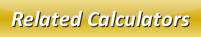# Multiplying Polynomials Calculator

Polynomial multiplication is easier when you learn it in a right way. While multiplying the polynomials, first you need to multiply the constants and then you need to multiply the variables. Let us learn it better with this simple below example:

Multiply the given polynomials (X+8)(X-5)

Solution:

Given: (X+8)(X-5)

Ā= XX + 8X - 5X - 5 x 8

Ā= X2 + 8X - 5X - 40

Ā= X2 + 3X - 40

Therefore, the answer is X2 + 3X - 40

Similarly, use our below online multiplying polynomials calculator to solve your problems instantly. Insert the polynomials into the respective input boxes and then click calculate button to know the answer.

Latest Calculator Release

Average Acceleration Calculator

Average acceleration is the object's change in speed for a specific given time period. ...

Free Fall Calculator

When an object falls into the ground due to planet's own gravitational force is known a...

Torque Calculator

Torque is nothing but a rotational force. In other words, the amount of force applied t...

Average Force Calculator

Average force can be explained as the amount of force exerted by the body moving at giv...

Angular Displacement Calculator

Angular displacement is the angle at which an object moves on a circular path. It is de...